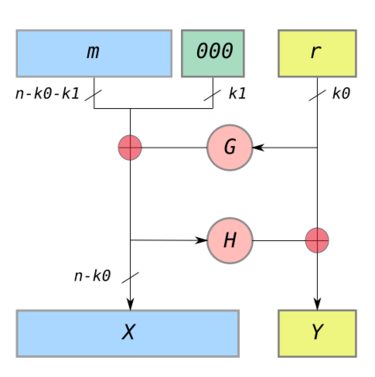Q

What is the OAEP padding schema used in OpenSSL "rsautl" command?

✍: FYIcenter.com

AOAEP (Optimal Asymmetric Encryption Padding), also called PKCS#1 2.0, is a padding standard specified in RFC3447 "PKCS #1: RSA Encryption, Version 1.5" proposed by RSA Laboratories in 1998.

OAEP padding can be illustrated by the diagram below:Here is the explanation of the above OAEP padding diagram given wikipedia.org:

```In the diagram,
n is the number of bits in the RSA modulus.
k0 and k1 are integers fixed by the protocol.
m is the plaintext message, an (n − k0 − k1 )-bit string
G and H are typically some cryptographic hash functions fixed by the protocol.
⊕ is an xor operation.

To encode,
messages are padded with k1 zeros to be n − k0 bits in length.
r is a randomly generated k0-bit string
G expands the k0 bits of r to n − k0 bits.
X = m00..0 ⊕ G(r)
H reduces the n − k0 bits of X to k0 bits.
Y = r ⊕ H(X)
The output is X || Y where X is shown in the diagram as the leftmost block
and Y as the rightmost block.

To decode,
recover the random string as r = Y ⊕ H(X)
recover the message as m00..0 = X ⊕ G(r)
```

Note that OAEP is not a simple padding by add some bytes before or after the clear text. OAEP will add bytes to the clear text and encode them to hide the clear text.